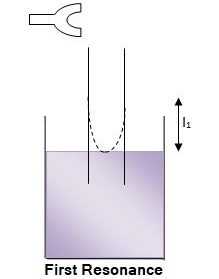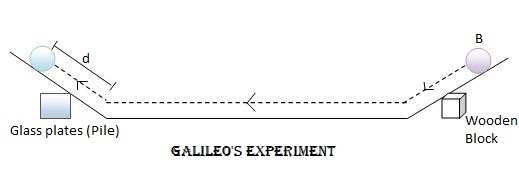## Random Posts## Vibrations of Air Column in Pipes

Investigatory Project – I

Vibrations of Air Column in Pipes – I

Aim: To study the factors affecting the resonant length of closed pipes.

Apparatus: Resonance column apparatus, Tuning forks, Rubber hammer, Metre scale etc.

Theory: In closed pipes, an antinode is formed at the open end and a node at the closed end. The distance between a node and the nearest antinode is λ/4. If ‘l’ is the length of the closed pipe, then l = λ/4.

Therefore, λ = 4l

The resonance occurs when the frequency of the tuning fork equals the frequency of natural vibrations of the closed pipe. Let v is the velocity of sound through air and n is the frequency of the tuning fork, then at resonance,

n = v/ λ = v/4l  or

l = v/4n

The velocity of sound is a constant at constant temperature.

Therefore, l 1/n or resonance length varies inversely with the frequency of the tuning fork.

Procedure:

Set the resonance column apparatus for experiment. Length of the air column is kept very small. A tuning fork of known frequency n, is excited and held horizontally near the mouth of the inner tube. The length of air column is measured. This is repeated again and the average length of the air column is determined. The experiment is repeated for tuning forks of different frequencies and the results are tabulated.

Observations and Calculations

Room temperature in the beginning = ____°C

Room temperature at the end = ____°C

 Frequency of the tuning fork (Hz) First resonating length Mean resonance length (cm) 1 (cm) 2 (cm)

Report:

At constant temperature the resonance length of closed pipes decreases with the increase in frequency of the driving source.

Investigatory Project – II

Vibrations of Air Column in Pipes – 2

Aim: To study the factors affecting the resonant length of closed pipe.

Apparatus: Resonance column apparatus, Tuning fork, Rubber hammer, Metre scale etc.

Theory: The wavelength (λ) corresponding to first resonating (l) is given by,

λ/4 = l +e, where end correction e = 0.3D, D is the inner diameter of the pipe.

The velocity of sound at room temperature (t) is v(t) = 4n(l+e) where n is the frequency of the tuning fork. At constant temperature, velocity of sound is a constant.

l 1/n

For constant n, λ is constant

Therefore, resonating length l (first resonating length observed) varies with diameter of the pipe.

Procedure:

Set the resonance column apparatus for experiment. Length of the air column is kept very small. A tuning fork of known frequency n, is excited and held horizontally over the mouth of the inner tube. The length of air column in the inner tube is slowly increased by raising the tube till a booming sound is heard. The length of the air column is measured. This is repeated again and the average length of the air column is determined. The experiment is repeated for pipes of different inner diameters.

Observations and Calculations

Frequency of the tuning fork, n = ___ Hz

Room temperature, t = ___°C

To study the vibration of resonating length with inner diameter of the pipe

 Trial No: Inner diameter of tube D (cm) First resonating length, l (cm) 1 2 3 4 5

Report:

The resonating length of closed pipe with its inner diameter at constant temperature is studied.## Galileo Experiment on Motion

Galileo Experiment on Motion Investigatory Project

Aim

To study the first law of motion by the double inclined track.

Apparatus

Double inclined track, Metal ball, Glass plate of same thickness, Wooden block etc. The double inclined track apparatus consists of a pair of inclined tracks, each of them can be independently set at a small angle of inclination.

Theory

A body will continue its state of rest or uniform motion along a straight line unless an external resultant force acts on it.

Procedure

Set up the double inclined track on the table. Clean the metal ball and the track using cotton or tissue paper. Insert the wooden block under the right arm at such a position that the track is inclined at a suitable angle (nearly 45°) with the horizontal. Then left arm is arranged at a suitable angle of inclination. This is done by placing a number of glass plates below it (say 14). A point 'B' is marked on the right arm which is at a distance 10 cm from the lower edge. The metal ball is released from 'B' and the distance 'd' to which it ascends in the left arm is noted. The experiment is repeated for different angle of inclinations in the left arm. This can be done by changing the number of glass plates below it. Since the glass plates are of same thickness, the slope of the left arm will be proportional to the number of glass plates (n).

A graph is plotted with number of glass plates (n) along the X-axis and the reciprocal of the distance 'd' along the Y-axis. The graph would be a straight line. On extrapolating the graph to slope zero (ie, n=0), it is found that 1/D is nearly zero or d = ∞, which implies that the body will continue its motion along a straight line. This verifies the validity of the first law of motion.

Observations and Calculations

 Trial No: of glass plates below the left arm (n) Distance of ascend along the left arm (d) 1/d 1 2 Mean (d) - - cm cm cm cm-1 1 2 3 4 5 6 14 12 10 8 6 4

Report

This experiment verifies that in the absence of an external force, a body in uniform motion would continue its motion indefinitely along a straight line.## Effect of particle size on rate of chemical reaction

Investigatory project on study of the effect of particle size on rate of chemical reaction

Principle

In reactions involving solids, the reaction usually takes place on the surface and the rate of the reaction depends on the surface area of the solid particles. The greater the surface area, the higher will be the rate of the reaction. The surface area of a given mass of solid depends on the size of the particle. For a given amount of a solid, the surface area increases with the decrease in size of the particle. So smaller the particles, greater will be the rate of reaction.

Objective

The objective of this project is to study the influence of the size of particles on the rate of a reaction by considering the reaction between marble and hydrochloric acid.

CaCO3 + 2HCl ---> CaCl2 + H2O + CO2

Requirements

250 mL beakers, Marble pieces, HCI acid, Stop watch

Procedure

Take one single piece of marble weighing 2g. Similarly take 2g samples of coarsely powdered marble and finely powdered marble. Place the three samples in three different beakers. Add the same quantity of 2M HCl (not less than 50 mL) to each of them. There should be enough acid to completely immerse the samples. As soon as HCI is added, start a stop watch. The contents may be shaken at regular intervals. Note the time taken in each case to complete the reaction. The reaction will be slowest when a single piece of marble is reacted. It is fastest when finely powdered sample is reacted.

 No Nature of marble (2g) Volume of 2M HCl in mL Time taken to complete the reaction 1 2 3 Fine powder Coarse powder Single piece 50 50 50 - - -

It is observed from the experiment that minimum time is taken for the completion of reaction when marble is in the powdered form.

Conclusion

The rate of reaction of a solid substance is proportional to the surface area of the sample.

1. How does the rate of a reaction vary with increase in surface area?

Ans: Rate of a reaction increases with increase in surface area

2. Why are powdered substances more reactive than substances in lump states?

Ans: Surface area of powdered substances are more than that of a substance in the lump state.

3. What is the action of dil.HCI on marble?

Ans: Marble reacts with HCl to liberate CO2.

4. Write the reaction between marble and HCl

Ans: CaCO3 + 2HCl ---> CaCl2 + H2O + CO2## Rate of Flow of Liquid Investigatory Project

Investigatory Project on comparative study of the rate of flow of liquids

Introduction: Liquids have flow properties. Some liquids flow faster than some others. This difference in the rate of flow of liquid is due to a property called viscosity. Viscosity of a liquid is its resistance to flow. A liquid with high viscosity will flow comparatively slowly. Viscosities of different liquids can be compared in terms of coefficient of viscosity. It is described as the force in dynes per square centimeter necessary to maintain a difference in velocity of 1 cm per second between two parallel layers of liquid held at a distance of 1cm from one another. It is denoted by η and expressed in millipoise. The rates of flow of liquids are compared by taking them in a viscometer and allowing them to flow through a fixed distance and measuring the time taken in each case.

Objective: The objective of this project is to compare the rates of flow of different liquids such as water, coconut oil, benzene, glycerine etc.

Requirements: Viscometer, Stop watch, Liquids like water, coconut oil, benzene, glycerine etc.

Procedure: Take one of the liquids, say water (about 25mL) in the bulb 'A' of a clean and dry viscometer. Suck up the liquid into bulb 'B' so that it is slightly above the mark 'C' of the viscometer. Allow the liquid to fall through the capillary. Note the time taken for the meniscus of the liquid to fall from the mark 'C' to 'D' by using a stop watch. Repeat the experiment three times with the same liquid. Wash and dry the viscometer. Repeat the above experiment with the other liquids and tabulate the results.

 Liquid Time taken Density d η liquid/ η water t1 t2 t3 Mean t Water - - - - Coconut oil - - - - Benzene - - - - Glycerine - - - -

η liquid/η water can be calculated using the relationship.

η liquid/η water = t liquid/t water x d liquid/d water

Where t liquid and t water are the time taken for the flow of liquid and water respectively and d liquid and d water are the densities of the liquid and water respectively.

Conclusion:

Liquids which flow readily have low viscosity, while those which flow slowly have high viscosity.

1. What is viscosity of a liquid?

Ans: Resistance to the flow of a liquid is known as viscosity.

2. Which has higher viscocity, water or coconut oil?

Ans: Coconut oil

3. Which instrument is used to measure viscosity?

Ans: Viscometer

4. Why is coconut oil more viscous than water?

Ans: Different liquid layers in coconut oil are attracted by hydrogen bonding.